# 报表的动态明细行的前端显示 C# ASP.net

## 简化的问题模型

students表：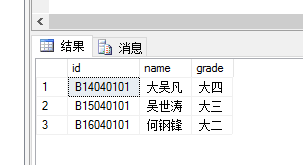scores表：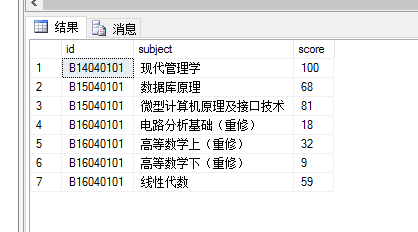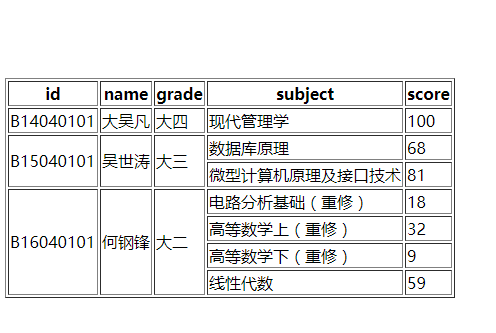## 分析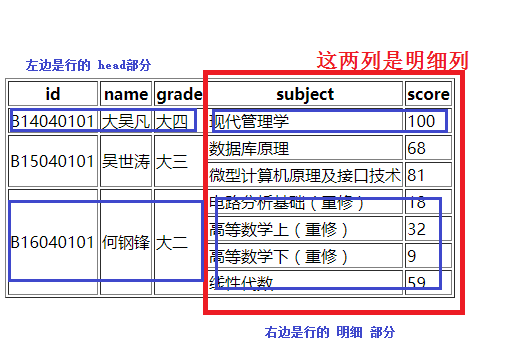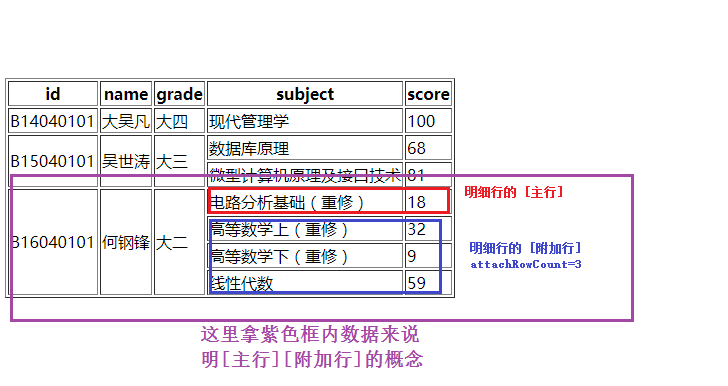<table><asp:Lable ...></table>


### 我们需要做的是拼接一个table内的字符串，放到这个lable里面就可以了。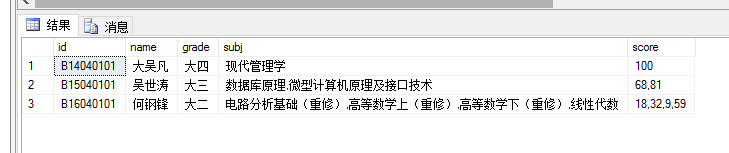http://blog.csdn.net/wf824284257/article/details/74905618

http://blog.csdn.net/wf824284257/article/details/76713400

    //该列是否是明细列
protected bool IsDetailCol(DataColumn dc)
{
switch(dc.ColumnName)
{
case "subject":
case "score":
return true;
default:
return false;
}
}


    //获取指定行的附加行数量。理清定义：若指定行明细数据有5个，则第一个算是主数据，后面4个算是附加行数据，所以返回4
protected int GetAttachRowCountByRow(DataRow dr)
{
int ans = 0;

string str = dr["subject"].ToString();
string[] strs = str.Split(',');
ans = strs.Length - 1;

return ans;
}


    //获取指定的明细数据
protected string GetAttachData(DataRow dr,DataColumn dc,int index)
{
//index: 逆序序号
string str = dr[dc].ToString();
string[] strs = str.Split(',');
int dataIndex = strs.Length - index;
return strs[dataIndex];
}


    //通常情况下：拼接<table></table>内的字符串
//protected string GetHtmlStrByDataTable(DataTable dt)
//{
//    StringBuilder sb = new StringBuilder();

//    //表头
//    sb.Append("<tr>");
//    foreach(DataColumn dc in dt.Columns)
//    {
//        sb.Append("<th>"+dc.ColumnName+"</th>");
//    }
//    sb.Append("</tr>");

//    //数据
//    for(int i=0;i<dt.Rows.Count;i++)
//    {
//        sb.Append("<tr>");
//        for(int j=0;j<dt.Columns.Count;j++)
//        {
//            sb.Append("<td>"+dt.Rows[i][j].ToString()+"</td>");
//        }
//        sb.Append("</tr>");
//    }

//    return sb.ToString();
//}


    /// <summary>
/// </summary>
/// <param name="dt"></param>
/// <returns></returns>
protected string GetHtmlStrByDataTable(DataTable dt)
{
StringBuilder sb = new StringBuilder();

//表头
sb.Append("<tr>");
foreach (DataColumn dc in dt.Columns)
{
sb.Append("<th>" + dc.ColumnName + "</th>");
}
sb.Append("</tr>");

//数据
int attachRowCount = 0;//当前行剩余附加行的数量
for (int i = 0; i < dt.Rows.Count; i++)
{
sb.Append("<tr>");

//正在处理行的明细部分：只对明细列进行html代码拼接
if(attachRowCount>0)
{
for (int j = 0; j < dt.Columns.Count; j++)
{
if (IsDetailCol(dt.Columns[j]))
{
sb.Append("<td>" + GetAttachData(dt.Rows[i], dt.Columns[j], attachRowCount) + "</td>");
}
}
attachRowCount--;//处理完一条明细
if (attachRowCount > 0) i--;//该行仍有明细数据
sb.Append("</tr>");
continue;
}

//正常处理行
attachRowCount = GetAttachRowCountByRow(dt.Rows[i]);

for (int j = 0; j < dt.Columns.Count; j++)
{
if(!IsDetailCol(dt.Columns[j]))
{
string tdRowSpan = "rowspan=\"" + (attachRowCount + 1).ToString() + "\" ";
sb.Append("<td " + tdRowSpan + ">" + dt.Rows[i][j].ToString() + "</td>");
}
else
{
sb.Append("<td>" + GetAttachData(dt.Rows[i],dt.Columns[j],attachRowCount+1)+ "</td>");
}
}
if(attachRowCount>0) i--;//该行仍有明细数据
sb.Append("</tr>");
}

return sb.ToString();
}


It’s a nice Day today.

07-3001-23109
06-271087
09-26
08-19
05-21737
12-224235
12-161684
05-178万+点击重新获取扫码支付余额充值